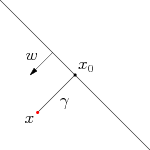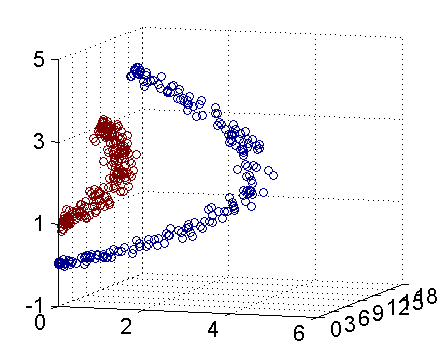# 【机器学习】支持向量机(SVM)

1.1 一个关于SVM的童话故事 支持向量机（Support Vector Machine，SVM）是众多监督学习方法中十分出色的一种，几乎所有讲述经典机器学习方法的教材都会介绍。关于SVM，流传着一个关于天使与魔鬼的故事。 传说魔鬼和天使玩了一个游戏，魔鬼在桌上放了两种颜色的球。魔鬼让天使用一根…

## 1. 讲讲SVM

### 1.1 一个关于SVM的童话故事SVM实际上是在为天使找到木棒的最佳放置位置，使得两边的球都离分隔它们的木棒足够远。依照SVM为天使选择的木棒位置，魔鬼即使按刚才的方式继续加入新球，木棒也能很好地将两类不同的球分开。### 1.2 理解SVM：第一层

$$w^Tx+b=0$$#### 1.2.1 函数间隔与几何间隔

$$\gamma=min\gamma i(i=1,…n)$$#### 1.2.2 最大间隔分类器的定义OK，到此为止，算是了解到了SVM的第一层，对于那些只关心怎么用SVM的朋友便已足够，不必再更进一层深究其更深的原理。

#### 1.2.3 最大间隔损失函数Hinge loss

SVM 求解使通过建立二次规划原始问题，引入拉格朗日乘子法，然后转换成对偶的形式去求解，这是一种理论非常充实的解法。这里换一种角度来思考，在机器学习领域，一般的做法是经验风险最小化 （empirical risk minimization,ERM），即构建假设函数（Hypothesis）为输入输出间的映射，然后采用损失函数来衡量模型的优劣。求得使损失最小化的模型即为最优的假设函数，采用不同的损失函数也会得到不同的机器学习算法。SVM采用的就是Hinge Loss，用于“最大间隔(max-margin)”分类。

$$L_i=\sum_{j\neq t_i}max(0,f(x_i,W)j-(f(x_i,W){y_i}-\bigtriangleup))$$

• 对于训练集中的第i个数据xi
• 在W下会有一个得分结果向量f(xi,W)
• 第j类的得分为我们记作f(xi,W)j1. 在生活中我们都会认为没有威胁的才是最好的，比如拿成绩来说，自己考了第一名99分，而第二名紧随其后98分，那么就会有不安全的感觉，就会认为那家伙随时都有可能超过我。如果第二名是85分，那就会感觉安全多了，第二名需要花费很大的力气才能赶上自己。拿这个例子套到上面这幅图也是一样的。
2. 上面这幅图delta左边的红点是一个安全警戒线，什么意思呢？也就是说预测错误得分超过这个安全警戒线就会得到一个惩罚权重，让这个预测错误值退回到安全警戒线以外，这样才能够保证预测正确的结果具有唯一性。
3. 对应到公式中，$f(x_i,W)_j$ 就是错误分类的得分。后面一项就是 正确得分 - delta = 安全警戒线值，两项的差代表的就是惩罚权重，越接近正确得分，权重越大。当错误得分在警戒线以外时，两项相减得到负数，那么损失函数的最大值是0，也就是没有损失。
4. 一直往复循环训练数据，直到最小化损失函数为止，也就找到了分类超平面。

### 1.3 深入SVM：第二层

#### 1.3.1 从线性可分到线性不可分

$$max\frac{1}{||w||}s.t.,y_i(w^Tx_i+b)\ge1,i=1,…,n$$

$$min\frac{1}{2}||w||^2s.t.,y_i(w^Tx_i+b)\ge1,i=1,…,n$$

#### 1.3.2 核函数Kernel1. 实际中，我们会经常遇到线性不可分的样例，此时，我们的常用做法是把样例特征映射到高维空间中去(映射到高维空间后，相关特征便被分开了，也就达到了分类的目的)；
2. 但进一步，如果凡是遇到线性不可分的样例，一律映射到高维空间，那么这个维度大小是会高到可怕的。那咋办呢？
3. 此时，核函数就隆重登场了，核函数的价值在于它虽然也是将特征进行从低维到高维的转换，但核函数绝就绝在它事先在低维上进行计算，而将实质上的分类效果表现在了高维上，避免了直接在高维空间中的复杂计算。

Linear Kernel线性核 $k(x,y)=x^{t}y+c$
Polynomial Kernel多项式核 $k(x,y)=(ax^{t}y+c)^{d}$ $d\geqslant1$为多项式的次数
Exponential Kernel指数核 $k(x,y)=exp(-\frac{\left |x-y \right |}{2\sigma ^{2}})$ $\sigma>0$
Gaussian Kernel高斯核 $k(x,y)=exp(-\frac{\left |x-y \right |^{2}}{2\sigma ^{2}})$ $\sigma$为高斯核的带宽，$\sigma>0$,
Laplacian Kernel拉普拉斯核 $k(x,y)=exp(-\frac{\left |x-y \right |}{\sigma})$ $\sigma>0$
ANOVA Kernel $k(x,y)=exp(-\sigma(x^{k}-y^{k})^{2})^{d}$
Sigmoid Kernel $k(x,y)=tanh(ax^{t}y+c)$ $tanh$为双曲正切函数，$a>0,c<0$

#### 1.3.3 总结

OK，理解到这第二层，已经能满足绝大部分人一窥SVM原理的好奇心，针对于面试来说已经足够了。

### 1.4 SVM的应用

SVM在很多诸如文本分类，图像分类，生物序列分析和生物数据挖掘，手写字符识别等领域有很多的应用，但或许你并没强烈的意识到，SVM可以成功应用的领域远远超出现在已经在开发应用了的领域。

## 2. SVM的一些问题

1. 是否存在一组参数使SVM训练误差为0？

答：存在

2. 训练误差为0的SVM分类器一定存在吗？

答：一定存在

3. 加入松弛变量的SVM的训练误差可以为0吗？

答：使用SMO算法训练的线性分类器并不一定能得到训练误差为0的模型。这是由于我们的优化目标改变了，并不再是使训练误差最小。

4. *带核的SVM为什么能分类非线性问题？ *

答：核函数的本质是两个函数的內积，通过核函数将其隐射到高维空间，在高维空间非线性问题转化为线性问题, SVM得到超平面是高维空间的线性分类平面。其分类结果也视为低维空间的非线性分类结果, 因而带核的SVM就能分类非线性问题。

5. 如何选择核函数？

• 如果特征的数量大到和样本数量差不多，则选用LR或者线性核的SVM；
• 如果特征的数量小，样本的数量正常，则选用SVM+高斯核函数；
• 如果特征的数量小，而样本的数量很大，则需要手工添加一些特征从而变成第一种情况。

## 3. LR和SVM的联系与区别

### 3.1 相同点

• 都是线性分类器。本质上都是求一个最佳分类超平面。
• 都是监督学习算法。
• 都是判别模型。判别模型不关心数据是怎么生成的，它只关心信号之间的差别，然后用差别来简单对给定的一个信号进行分类。常见的判别模型有：KNN、SVM、LR，常见的生成模型有：朴素贝叶斯，隐马尔可夫模型。

### 3.2 不同点

• LR是参数模型，svm是非参数模型，linear和rbf则是针对数据线性可分和不可分的区别；
• 从目标函数来看，区别在于逻辑回归采用的是logistical loss，SVM采用的是hinge loss，这两个损失函数的目的都是增加对分类影响较大的数据点的权重，减少与分类关系较小的数据点的权重。
• SVM的处理方法是只考虑support vectors，也就是和分类最相关的少数点，去学习分类器。而逻辑回归通过非线性映射，大大减小了离分类平面较远的点的权重，相对提升了与分类最相关的数据点的权重。
• 逻辑回归相对来说模型更简单，好理解，特别是大规模线性分类时比较方便。而SVM的理解和优化相对来说复杂一些，SVM转化为对偶问题后,分类只需要计算与少数几个支持向量的距离,这个在进行复杂核函数计算时优势很明显,能够大大简化模型和计算。
• logic 能做的 svm能做，但可能在准确率上有问题，svm能做的logic有的做不了。

## 4. 线性分类器与非线性分类器的区别以及优劣

• 线性分类器可解释性好，计算复杂度较低，不足之处是模型的拟合效果相对弱些。

LR,贝叶斯分类，单层感知机、线性回归

• 非线性分类器效果拟合能力较强，不足之处是数据量不足容易过拟合、计算复杂度高、可解释性不好。

决策树、RF、GBDT、多层感知机

SVM两种都有（看线性核还是高斯核）